Courses

# Mathematics Notes | EduRev

## Mathematics: Mathematics Notes | EduRev

The document Mathematics Notes | EduRev is a part of the Mathematics Course Algebra for IIT JAM Mathematics.
All you need of Mathematics at this link: Mathematics

In this final section of this chapter we are going to look at another series representation for a function.  Before we do this let’s first recall the following theorem.

Binomial Theorem
If n is any positive integer then,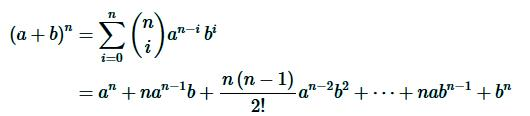where,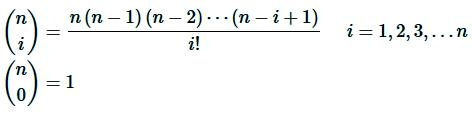This is useful for expanding (a+b)n for large n when straight forward multiplication wouldn’t be easy to do.  Let’s take a quick look at an example.

Example 1 Use the Binomial Theorem to expand (2x−3)4

Solution. There really isn’t much to do other than plugging into the theorem.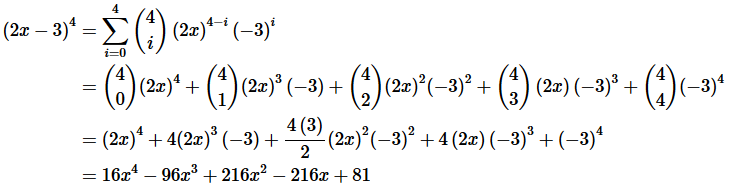Now, the Binomial Theorem required that n be a positive integer.  There is an extension to this however that allows for any number at all.

Binomial Series

If k is any number and |x|<1 then,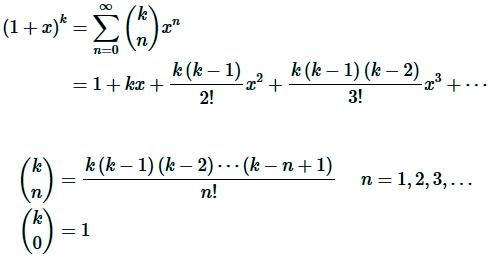So, similar to the binomial theorem except that it’s an infinite series and we must have |x<1 in order to get convergence.
Let’s check out an example of this.

Example 2 Write down the first four terms in the binomial series for  √9−x

Solution. So, in this case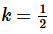and we’ll need to rewrite the term a little to put it into the form required.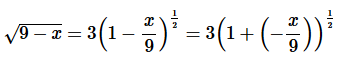The first four terms in the binomial series is then,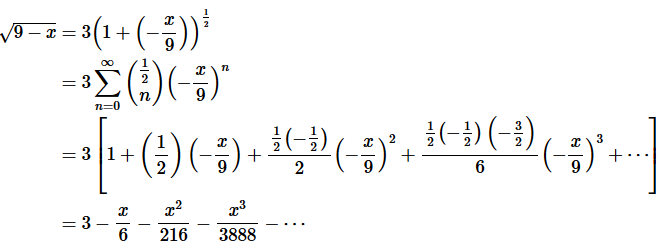The document Mathematics Notes | EduRev is a part of the Mathematics Course Algebra for IIT JAM Mathematics.
All you need of Mathematics at this link: MathematicsUse Code STAYHOME200 and get INR 200 additional OFF Use Coupon Code
All Tests, Videos & Notes of Mathematics: Mathematics## Algebra for IIT JAM Mathematics

70 videos|58 docs

Track your progress, build streaks, highlight & save important lessons and more!

,

,

,

,

,

,

,

,

,

,

,

,

,

,

,

,

,

,

,

,

,

;